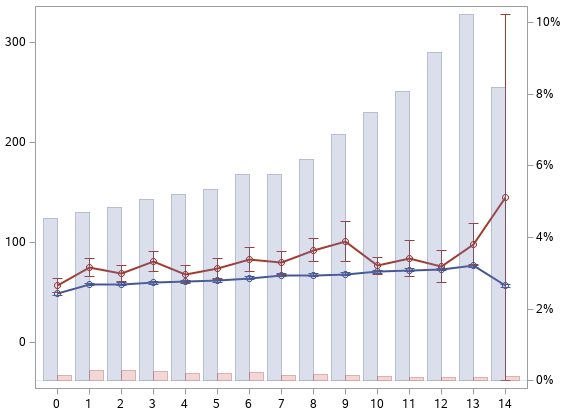## How do I get percents by group in SGPLOT?

I am using this code to get percent of bars within group (data_column_C), but what I am getting is percent within data_column_A category.

Basically I want the percents to sum up to 100% for data_column_C and have individual bar by data_column_A.

``````proc sgplot data=data_table pctlevel=group;
vline data_column_A /response=data_column_B stat=mean limitstat=clm markers group=data_column_C lineattrs=(thickness=2);
vbar data_column_A / response=data_column_B y2axis transparency=0.75 group=data_column_C groupdisplay=cluster stat=percent;
run;``````
10 REPLIES 10DanH_sas
SAS Super FREQ

## Re: How do I get percents by group in SGPLOT?

If I'm interpreting your request correctly, you really want to set PCTLEVEL=GRAPH (which is the default). Give that a try and see if the numbers are what you expect.

Thanks!

Dan

## Re: How do I get percents by group in SGPLOT?

I had tried that, when I do PCTLEVEL=GRAPH I get percents for the entire data.

If I do PCTLEVEL=GRAPH and then add a BY DATA_COLUMN_C statement after VBAR, then I get what I need but in two different graphs. But I am trying to have them both in one GRAPH.

Trying to get distribution of data in PERCENT but the percents should add up to 100 by the GROUP variable.DanH_sas
SAS Super FREQ

## Re: How do I get percents by group in SGPLOT?

Can you post a picture of what you're getting without the BY statement?

## Re: How do I get percents by group in SGPLOT?

What I want is that the blue bars sum up to 100% and the pink bars also sum up to 100%. The variable name differentiating them is DATA_COLUMN_C in my code above. 0-14 are DATA_COLUMN_A values and DATA_COLUMN_B is the response variable.DanH_sas
SAS Super FREQ

## Re: How do I get percents by group in SGPLOT?

The way you can do this is to transpose the data such that the values in DATA_COLUMN_B are put into DATA_COLUMN_B1 and DATA_COLUMN_B2, depending on the value in DATA_COLUMN_C. Then, your plot becomes 2 vbar sttements with stat=mean and 2 vline statements with stat=percent. The PCTLEVEL option will not be required.

Hope this helps!

Dan

## Re: How do I get percents by group in SGPLOT?

In this case I have 2 distinct values in DATA_COLUMN_C, but I more in other scenarios. So transforming and repeating should work as you suggested, but I wish there was an option that would make it happen without transforming data. From the documentation PCTLEVEL seemed like was the solution but it is giving percent by DATA_COLUMN_A grouping. Thanks for your responses, will have to do that work around if no other solution.

## Re: How do I get percents by group in SGPLOT?

i think this is a much needed function in sgplot.

my work-around is to use proc freq to export the percentages and then use sgplot. like

proc freq data=xxx noprint;table a*b/out=XXXX outpct;run;

proc sgplot data=XXXX;vbar b /response=pct_row group=a groupdisplay=cluster;run;

## Re: How do I get percents by group in SGPLOT?

This exact code worked for me. Thank you.

## Re: How do I get percents by group in SGPLOT?

These work around codes work for me. Now, I'm having the percentage display on the bars (datalabel). Is it possible to limit the decimal places to one only. It's displaying 3 and that's too much.

Thanks.

## Re: How do I get percents by group in SGPLOT?

This is exactly what I'm trying to do! But it looks like proc sgplot doesn't offer any options.
Discussion stats
• 10 replies
• 4928 views
• 6 likes
• 6 in conversation